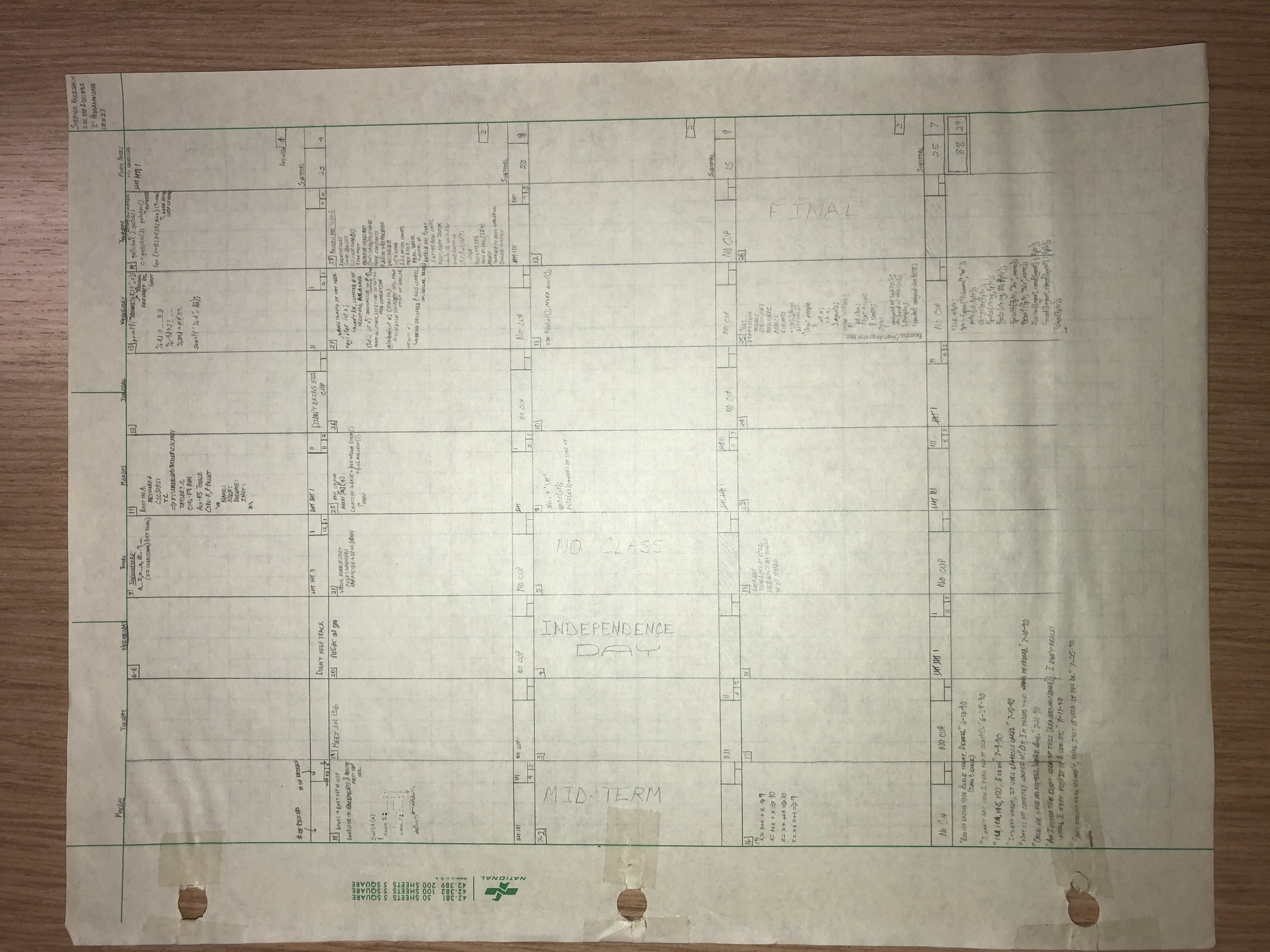weight = 34

# Self-Learning Module

## Outcomes Covered

### More Standard Java Classes

• Define an Application Programming Interface (API)
• Use Oracle's Java documentation to ascertain the capabilities of a given standard java class
• Use the Javadoc page for the Math class to perform calculations involving the following mathematic operations:
• Absolute value
• Trigonometric functions (in degrees and radians)
• pi - ratio of the circumference of a circle to its diameter
• xy
• logarithmic functions
• maximum/minimum of two numbers
• Square root
• Generate random numbers

## Math Class [4:57]

• Study sections 5.1-3 of the textbook
• Watch the following video [link]
• You should be able to answer questions 1-5 of the Self-Learning Module 4 quiz in Canvas.

## Math Exercises

Complete the following challenges on your own (in IntelliJ) to become familiar with the methods in the Math class.

• Determine the larger of two numbers without using an `if` statement.
• Determine the absolute value of an `int`.
• Determine the absolute value of an `double`.
• Determine the sine of `45` degrees.
• Determine the tangent of `0.5` in radians.
• Determine the value of `3``5`.
• Determine the closest integer value to `8.87`.

## Generating Random Numbers [6:02]

• Watch the following video [link]
• You should be able to answer questions 6-10 of the Self-Learning Module 4 quiz in Canvas.

# Bonus Material (optional)

• Sabrina Stangler on aysnchronous videos [1:19] [link]
• Dr. Taylor on tricks to stay focused [2:14] [link]